﻿ 基于Cox比例风险回归模型、LASSO与生存树的乳腺癌预后 Prognosis of Breast Cancer Based on Cox Proportional Hazards Regression Model, LASSO and Survival Tree

Statistics and Application
Vol.07 No.02(2018), Article ID:24328,12 pages
10.12677/SA.2018.72013

Prognosis of Breast Cancer Based on Cox Proportional Hazards Regression Model, LASSO and Survival Tree

Li Wang, Juan Zhang

North China Electric Power University, Beijing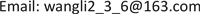Received: Mar. 13th, 2018; accepted: Apr. 1st, 2018; published: Apr. 8th, 2018ABSTRACT

Traditional pathological examination methods are not enough to predict the treatment outcome of breast cancer. Therefore, it is of great significance to study the pathogenesis of breast cancer by molecular biology. By predicting the risk of recurrence in patients with breast cancer, high-risk cancer patients can benefit from adjuvant therapy, while low-risk cancer patients can be protected from unnecessary treatment. The microarray data of ER+ breast cancer and ER− breast cancer were analyzed in this paper. Univariate Cox proportional hazards regression mode was used to preliminary screening the genes, then the LASSO was further used to screen the genes and applied the genes to the survival tree for prediction and classification, Kaplan-meier curve and log-rank test were used to prove the validity of the result. The model in this paper has a good prediction effect in the classification of breast cancer patients. Some of the genes we screened have been reported in the relevant literature, indicating that it is closely related to the occurrence and development of breast cancer. Other genes need further experiments to verify the role they play in breast cancer.

Keywords:Cox Proportional Hazards Regression Model, Kaplan-Meier Curve, Log-Rank Test, LASSO, Survival Tree1. 引言

2. 研究对象和方法

2.1. 研究对象

2.2. 研究方法

3. 模型建立

3.1. 数据预处理Table 2. The basic information of breast cancerTable 3. The matching table of probes and genesTable 4. The encoding matrix of ER+ breast cancerTable 5. The encoding matrix of ER− breast cancer

3.2. 单因素Cox比例风险回归模型初步筛选基因

Cox比例风险回归模型  是由英国的生物统计学家Cox D R提出的比例风险模型。风险函数(hazard function)是描述生存时间分布的一个重要函数。如终点事件为死亡(复发)，风险函数表示t时刻仍存活的病人在t时刻的瞬间死亡(复发)率：

$h\left(t\right)=\underset{\Delta t\to 0}{\mathrm{lim}}\frac{在时间t生存的病人死\left(复发\right)于区间\left(t,t+\Delta t\right)的概率}{\Delta t}$

Cox提出的比例风险模型是：病人具有 ${X}_{i1},{X}_{i2},\cdots ,{X}_{ip}$ 的伴随变量值，则第i名病人生存(复发)到时间t的风险函数是基础风险函数与自变量的函数的乘积：

${h}_{i}\left(t\right)={h}_{0}\left(t\right)×f\left({\beta }_{1}{X}_{i1}+\cdots +{\beta }_{p}{X}_{ip}\right)$

${h}_{0}\left(t\right)$ 即当所有的伴随变量都为0时的风险函数。其中定义伴随变量的函数 $f\left({x}_{ij}\beta \right)$ 为指数形式，因此

${h}_{i}\left(t\right)={h}_{0}\left(t\right)×\mathrm{exp}\left({\beta }_{1}{X}_{i1}+\cdots +{\beta }_{p}{X}_{ip}\right)$

$\mathrm{ln}\frac{{h}_{i}\left(t\right)}{{h}_{0}\left(t\right)}={\beta }_{1}{X}_{i1}+\cdots +{\beta }_{p}{X}_{ip}$

Cox模型是一个风险比对数的线性模型， ${\beta }_{j}$ 实际意义为当伴随变量 ${X}_{j}$ 每改变一个单位时所引起的相对风险度的自然对数的改变量。 ${\beta }_{j}$ 不仅反映出协变量的作用强度，而且反映它的作用方向  。Table 6. Primary screening genes by univariate Cox proportional hazards regression model (ER+)Table 7. Primary screening genes by univariate Cox proportional hazards regression model (ER−)

3.3. 计算风险分数，对患者进行分类Table 8. Calculate the risk scores and classify the ER+ breast cancer of training setTable 9. Calculate the risk scores and classify the ER− breast cancer of training set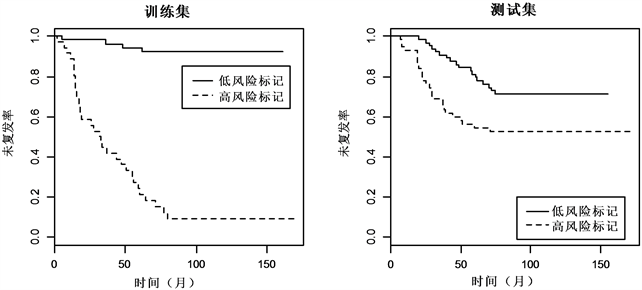Figure 1. Kaplan-Meier estimates of survival of ER+ breast cancer according to the 999-gene signatures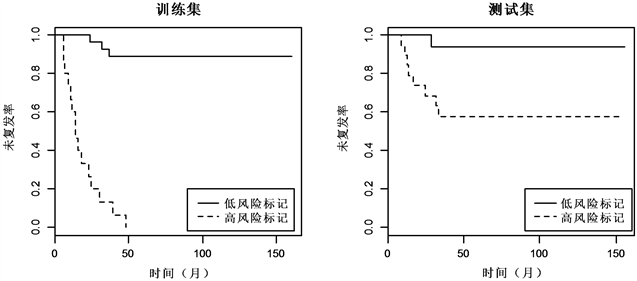Figure 2. Kaplan-Meier estimates of survival of ER− breast cancer according to the 13-gene signatures

3.4. LASSO方法筛选基因

$\underset{\beta }{\mathrm{min}}\sum _{i=1}^{n}{\left({y}_{i}-\sum _{j=1}^{p}{\beta }_{j}{x}_{ij}\right)}^{2}\text{\hspace{0.17em}},\text{\hspace{0.17em}}\text{ }\text{subject}\text{\hspace{0.17em}}\text{to}\text{ }\text{\hspace{0.17em}}\text{\hspace{0.17em}}\sum _{j=1}^{p}|{\beta }_{j}|\le \lambda$

$\stackrel{^}{\beta }\left(\text{Lasso}\right)=\mathrm{arg}\text{\hspace{0.17em}}\mathrm{min}\left\{{\sum _{i=1}^{n}‖{y}_{i}-\sum _{j=1}^{p}{x}_{ij}{\beta }_{j}‖}^{2}+\lambda \sum _{j=1}^{p}|{\beta }_{j}|\right\},\text{\hspace{0.17em}}\text{\hspace{0.17em}}\lambda 为调整参数$

3.5. 生存树方法进行预测

$G\left({s}^{*},h\right)=\underset{s\in {s}_{h}}{\mathrm{max}}G\left(s,h\right)$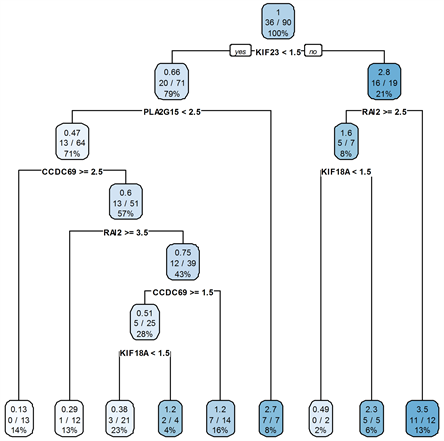Figure 3. Survival tree of ER+ breast cancer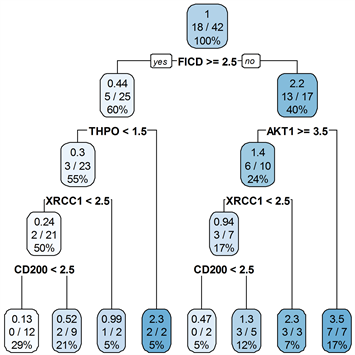Figure 4. Survival tree of ER− breast cancer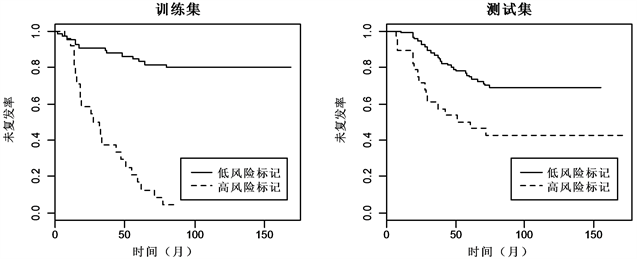Figure 5. Kaplan-Meier estimates of survival of ER+ breast cancer according to the 5-gene signatures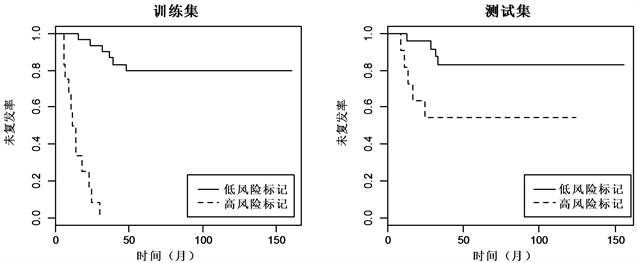Figure 6. Kaplan-Meier estimates of survival of ER− breast cancer according to the 5-gene signatures

4. 讨论

1. CCDC69、KIF18A、KIF23、PLA2G15、RAI2对ER+乳腺癌的复发具有显著性影响；

2. AKT1、CD200、FICD、THPO、XRCC1对ER−乳腺癌的复发具有显著性影响。

CCDC69、KIF23、PLA2G15这3个基因在ER+乳腺癌中发挥的作用及FICD和THPO在ER−乳腺癌中发挥的作用仍需通过进一步生物实验验证，其可能为ER+乳腺癌和ER−乳腺癌复发的潜在影响因子。总之，由我们筛选的基因对ER+与ER−乳腺癌患者风险高低的分类与患者临床结果密切相关。希望这些基因能为进一步实验提供理论依据，以研究这些基因在乳腺癌的预后中发挥的潜在作用。

Prognosis of Breast Cancer Based on Cox Proportional Hazards Regression Model, LASSO and Survival Tree[J]. 统计学与应用, 2018, 07(02): 99-110. https://doi.org/10.12677/SA.2018.72013

1. 1. 蒋定锋, 高峻, 赵耐青. 乳腺癌基因芯片数据分析[J]. 复旦学报(医学版), 2005, 32(2): 169-172.

2. 2. Higa, G.M. and Fell, R.G. (2013) Sex Hormone Receptor Repertoire in Breast Cancer. International Journal of Breast Cancer, 2013, 284036.

3. 3. 陈慧, 莫淋, 徐晓帆, 等. 雌激素受体阳性乳腺癌预后的相关因素分析[J]. 临床肿瘤学杂志, 2015, 20(4): 333-337.

4. 4. 刘宁. 乳腺癌基因分型的研究进展[J]. 中国普通外科杂志, 2010, 19(5): 556-559.

5. 5. Bolstadm B,M,, Irizarrym R,A,, Astrandm M,, et al. (2003) A Comparison of Normalization Methods for High Density Oligonucleotide Array Data Based on Variance and Bias. Bioinformatics, 19, 185-193. https://doi.org/10.1093/bioinformatics/19.2.185

6. 6. Hsiao, L.L. (2007) A Five-Gene Signature and Clinical Outcome in Non-Small-Cell Lung Cancer. New England Journal of Medicine, 356, 11. https://doi.org/10.1056/NEJMoa060096

7. 7. Cox, D.R. (1992) Regression Models and Life-Tables. Breakthroughs in Statistics. Springer, New York, 187-220. https://doi.org/10.1007/978-1-4612-4380-9_37

8. 8. 李元章, 何春雄. 实用生存模型: 不完全数据分析[M]. 广州: 华南理工大学出版社, 2015: 95-103.

9. 9. Warnke, R. (2004) Prediction of Survival in Diffuse Large-B-Cell Lymphoma Based on the Expression of Six Genes. The New England Journal of Medicine, 350, 1828-1837. https://doi.org/10.1056/NEJMoa032520

10. 10. Beer, D.G., Kardia, S.L., Huang, C.C., et al. (2006) Gene-Expression Profiles Predict Survival of Patients with Lung Adenocarcinoma. The Journal of Evidence-Based Medicine, 8, 816-824.

11. 11. Tibshirani, R. (1997) The Lasso Method for Variable Selection in the Cox Model. Statistics in Medicine, 16, 385-395. 3.0.CO;2-3>https://doi.org/10.1002/(SICI)1097-0258(19970228)16:4<385::AID-SIM380>3.0.CO;2-3

12. 12. 闫丽娜, 覃婷, 王彤. LASSO方法在Cox回归模型中的应用[J]. 中国卫生统计, 2012, 29(1): 58-60, 64.

13. 13. Simon, N., Friedman, J., Hastie, T., et al. (2011) Regularization Paths for Cox’s Proportional Hazards Model via Coordinate Descent. Journal of Statistical Software, 39, 1. https://doi.org/10.18637/jss.v039.i05

14. 14. Gordon, L. and Olshen, R.A. (1985) Tree-Structured Survival Analysis. Cancer Treatment Reports, 69, 1065-1069.

15. 15. 郎素平, 余红梅, 王彤, 等. 生存树方法及其在预后分析中的应用[J]. 中国卫生统计, 2006, 23(1): 13-15.

16. 16. Atkinson, E.J. and Therneau, T.M. (2000) An Introduction to Recursive Partitioning Using the RPART Routines. Rochester Mayo Foundation.

17. 17. Zhang, C., Zhu, C., Chen, H., et al. (2010) Kif18A Is Involved in Human Breast Carcinogenesis. Carcinogenesis, 31, 1676-1684. https://doi.org/10.1093/carcin/bgq134

18. 18. Werner, S., Borgmann, K., Pantel, K., et al. (2016) Abstract 2733: Novel Function of the RAI2 Protein in Genomic Integrity of Breast Cancer Cells. Cancer Research, 76, 2733-2733.

19. 19. Kabraji, S., Sole, X., Ying, H., et al. (2017) AKT1low Quiescent Cancer Cells Persist after Ne-oadjuvant Chemotherapy in Triple Negative Breast Cancer. Breast Cancer Research, 19, 88.

20. 20. Spears, M., Cunningham, C.A., Taylor, K.J., et al. (2012) Proximity Ligation Assays for Isoform-Specific Akt Activation in Breast Cancer Identify Activated Akt1 as a Driver of Progression. Journal of Pathology, 227, 481-489. https://doi.org/10.1002/path.4022

21. 21. Erin, N., Podnos, A., Tanriover, G., et al. (2015) Bidirectional Effect of CD200 on Breast Cancer Development and Metastasis, with Ultimate Outcome Determined by Tumor Aggressiveness and a Cancer-Induced Inflammatory Response. Oncogene, 34, 3860-3870. https://doi.org/10.1038/onc.2014.317

22. 22. Moullan, N., Cox, D.G., Angele, S., et al. (2003) Polymorphisms in the DNA Repair Gene XRCC1, Breast Cancer Risk, and Response to Radiotherapy. Cancer Epidemiology, Biomarkers & Prevention, 12, 1168-1174.